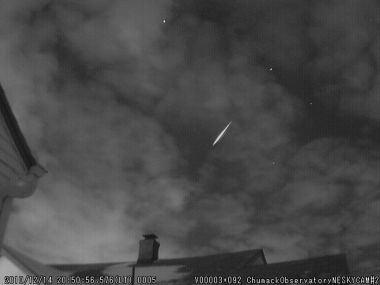# Falling MeteorA meteor of initial mass $m_{0}$ enters the earths atmosphere and starts falling from rest under the influence of gravity. Assume that the meteor gains mass from the cloud at a rate proportional to the momentum of the meteor, $\frac { d{ m }_{ 0 } }{ dt } =k{ m }_{ 0 }v$ , where $v$ instantaneous velocity of the meteor. If $k$ is equal to $5 m^{-1}$, what will be the terminal velocity of the meteor?

Take into consideration the buoyant force of the cloud

Details and assumption

You may neglect air resistance.

Take gravitational acceleration $9.8m/{ s }^{ 2 }$

×

Problem Loading...

Note Loading...

Set Loading...